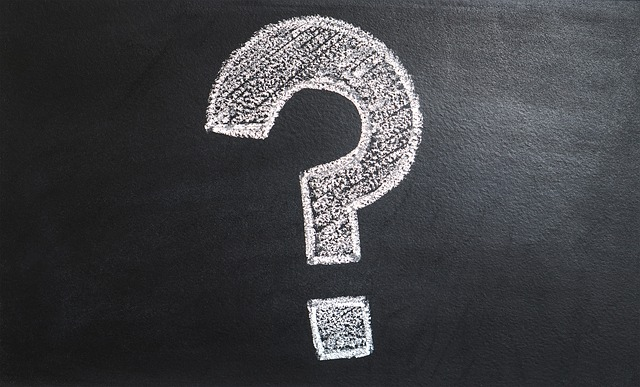# Lower-Level Vs Higher-Level Questions

Using Blooms Taxonomy of Educational Objectives, we can classify questions into two categories. These are lower-level and higher-level questions.

### What’s the Difference?To understand the difference between these two types of questions, let’s have a short review of Bloom’s Taxonomy. There are six general levels in Bloom’s Taxonomy:

• Remember (Knowledge)
• Understand (Comprehension)
• Apply (Application)
• Analyze (Analysis)
• Create (Synthesis)
• Evaluate (Evaluation)

Related: How to Use Questions in Class

The first level, Remember, is the lowest of level of expertise. All the student has to do is to recall the information provided from a source to prove that he or she is in this level. Learning in school usually starts in this level. As the student engages in more learning activities, he or she achieves objectives in the higher levels.

By studying the lesson, a student may achieve the objective of understanding the lesson. This falls in the second level of Bloom’s Taxonomy, Understand. In this stage, the student, doesn’t merely recall the information. He also understands relationships of the information he gained from previously learned concepts.

This continues until the last levels of expertise, where in the student is able to put learned conceptual elements together to Create and Evaluate.

### The Role of Questions in Bloom’s Taxonomy

The teacher can use questions to explore the levels of expertise of the students. They can be used in the beginning when the teacher is diagnosing the skill level of the students.

Questions could also be used during class as a part of the activity. A teacher for example, can use questions to keep class discussions going. Questions used in this way allows the students to participate in the class. It also prevents that teacher from dominating the discussion.

Lastly, questions can be used in the end part of the lesson to assess the learning of the students. They can be used to derive objective answers (i.e. multiple choice questions) from students as well as conceptual answers (i.e. essay questions).

### Low-Level Questions

The types of questions used in these activities are considered low-level questions when they cater to the first three levels of Bloom’s taxonomy (Remember, Understand, and Apply).

Most objective questions are considered low level questions because they only encourage the students to remember a certain information. This includes questions that start with:

• What is…?
• Who is…?
• Where did…?

These are the types of questions you usually see in the tests of young students.

Questions that ask for an explanation, fall under the second level (Understand). Questions in this level of expertise may start with the following phrases:

• How would you explain…?
• Why is the…?
• How does photosynthesis happen?

These types of questions checks if the student understands the concept or not. It is a step above just remembering information.

Application questions are also considered low level questions. They ask the student how a certain task or process is done. Here are some examples of questions in the application level:

• What is the process we use to solve for X?
• What would you say in an interview if you were asked…?

The goal here is to solve a problem using methods, processes or techniques defined in the lesson.

### Higher-level Questions

Higher level questions are those that seek answers in the last three levels of Bloom’s Taxonomy (Analyze, Evaluate, and Create).

In the analysis level, the teacher seeks to find out if the students are able to break down a concept, idea, process or thing into parts. The teacher also wants to find out if the student are able to relate these parts into their function to the whole.

Here are some examples of analysis questions:

• How is the keyboard important in the computer system?
• What were Romeo’s goals and how did they affect the plot of the story?
• What is the role of carbon dioxide in the photosynthetic process?

Evaluation, requires the students to develop their own judgments based on specific criteria or standards. Questions in this level are more open-ended. They give more room for student input, making them more difficult to answer.

Examples of questions in this level are:

• What do you think about democracy in relation to communism?
• Which is a better method of solving this math problem, solution 1 or solution 2?
• What is your stand on the Reproductive Health Bill and why?

The last level in Bloom’s taxonomy is the Creation stage. In this level of expertise, the student should be able to use the concepts he or she learned to create solutions, things, processes and other products of knowledge.

Here are some examples of questions in this level:

• If you were the author, how would you write the ending of this story?
• What would happen if you add carbon dioxide to the mixture?
• How would you design a marketing campaign that would sell this product?

More Prof. Ed. Notes: Professional Education Reviewer

### LET Questions about Low Level and High Level Questions:

1. Which is an example of a high level question?

a. What do you call words that represent actions?
b. Who is the protagonist in Florante and Laura?
c. What are the stages of cell division?
d. What factors in the Philippine economy are affecting inflation?

2. “How would you proceed if you were going to do an experiment on caloric intake?”
This is an example of a question in which level of Bloom’s Taxonomy?

a. Comprehension
b. Analysis
c. Evaluation
d. Synthesis

3. “What is the definition of “verb”?”
What type of question is this?

a. Probing Question
b. High level Question
c. Low Level Question
d. Analytical Question